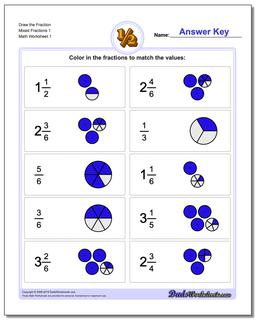# Math Worksheets: Graphic Fractions: Graphic Fractions: Draw the Fraction Mixed Fractions 1## Draw the Fraction Mixed Fractions 1

PropertyValue
DescriptionDraw the Fraction Mixed Fractions 1: For these problems, kids are given a simple fraction and asked to draw a pie chart representation of the value completely independently; unlike the worksheets from the prior section, these worksheets do not include the pre-divided fraction shapes as hints. Initial worksheets start out with basic fractions, and later sheets introduce mixed fractions.
Resource TypeWorksheet